# 2 Measurement Process Characterization

## 2.1 Packages used in this chapter

library(magrittr)
##
## Attaching package: 'magrittr'
## The following object is masked from 'package:purrr':
##
##     set_names
## The following object is masked from 'package:tidyr':
##
##     extract
library(tidyverse)

## 2.4 Case Studies

### 2.4.1 Check standard

#### 2.4.1.1 Background and data

The measurements on the check standard duplicate certification measurements that were being made, during the same time period, on individual wafers from crystal #51939. For the check standard there were:

• J = 6 repetitions at the center of the wafer on each day
• K = 25 days

Check standard for resistivity measurement

#### 2.4.1.2 Reading the dataset

check_standard <- read_table2("NIST data/MPC62_clean.DAT", col_names = TRUE) %>%
rowid_to_column()
## Parsed with column specification:
## cols(
##   Crystal_ID = col_integer(),
##   Wafer_ID = col_integer(),
##   Month = col_character(),
##   Day = col_character(),
##   Hour = col_character(),
##   Minute = col_character(),
##   Operator = col_integer(),
##   Humidity = col_integer(),
##   Probe_ID = col_integer(),
##   Temperature = col_double(),
##   Resistance = col_double(),
##   Stdev = col_double(),
##   Df = col_integer()
## )
check_standard
## # A tibble: 25 x 14
##    rowid Crystal_ID Wafer_ID Month Day   Hour  Minute Operator Humidity
##    <int>      <int>    <int> <chr> <chr> <chr> <chr>     <int>    <int>
##  1     1      51939      137 03    24    18    01            1       42
##  2     2      51939      137 03    25    12    41            1       35
##  3     3      51939      137 03    25    15    57            1       33
##  4     4      51939      137 03    28    10    10            2       47
##  5     5      51939      137 03    28    13    31            2       44
##  6     6      51939      137 03    28    17    33            1       43
##  7     7      51939      137 03    29    14    40            1       36
##  8     8      51939      137 03    29    16    33            1       35
##  9     9      51939      137 03    30    05    45            2       32
## 10    10      51939      137 03    30    09    26            2       33
## # ... with 15 more rows, and 5 more variables: Probe_ID <int>,
## #   Temperature <dbl>, Resistance <dbl>, Stdev <dbl>, Df <int>

#### 2.4.1.3 Level-1 standard deviation

Measurements for J runs over K days for L runs are:

$Y_{lkj}(l = 1, \, \ldots, \, L, \,\, k=1, \,\ldots, \, K, \,\, j=1, \,\ldots, \, J)$ The level-1 repeatability (short term precision) is calcuated from the pooled standard deviation over days and runs

$s_{1lk} = \sqrt{\frac{1}{J-1} \sum_{j=1}^{J}{(Y_{lkj} - \overline{Y}_{lk{\small \, \bullet}})^2}}$

with

$\overline{Y}_{lk{\small \, \bullet}} = \frac{1}{J}\sum_{j=1}^{J}{\overline{Y}_{lkj}}$ As stated in the e-Handbook: >An individual short-term standard deviation will not be a reliable estimate of precision if the degrees of freedom is less than ten, but the individual estimates can be pooled over the K days to obtain a more reliable estimate.

The pooled level-1 standard deviation estimate with v = K(J - 1) degrees of freedom is $s_1 = \sqrt{\frac{1}{K} \sum_{k=1}^{K} s_k^2}$

s1_chkstd <- check_standard %>%
mutate(Stdev_sq = Stdev^2) %$% mean(Stdev_sq) %>% sqrt() s1_chkstd ##  0.06138795 Several comments on the code above. I’ve introduced the %$% operator. This allows me to use indivdual columns from my data frame and is useful for preforming mathematical operations on a specific column of data. It is from the magrittr package.

I find this type of code easy to read and understand. Describing the operations is simple, I’m just working from inside out of the equation:

• creating a new column of data that is $$(Stdev)^2$$
• finding the mean of that new column
• taking the square root of that number to give $$s_1$$.

#### 2.4.1.4 Level-2 standard deviation (reproducibility)

$s_{chkstd} = {\large s}_2 = \sqrt{\frac{1}{K-1} \sum_{k=1}^{K} \left( \overline{Y}_{k \, \small{\bullet}} - \overline{Y}_{\small{\bullet} \small{\bullet}} \right) ^2}$ with

$\overline{Y}_{\small{\bullet} \small{\bullet}} = \frac{1}{K} \sum_{k=1}^{K} \overline{Y}_{k \, \small{\bullet}}$

Which is simply the standard deviation of the daily measuremnts

s2_chkstd <- check_standard %$% sd(Resistance) s2_chkstd ##  0.02679813 #### 2.4.1.5 Control chart for standard deviation - Precision UCL_precision_ckkstd <- s1_chkstd*sqrt(qf(0.95, 5, 125)) UCL_precision_ckkstd ##  0.0928313 ggplot(check_standard) + geom_point(aes(rowid, Stdev)) + geom_hline(aes(yintercept = UCL_precision_ckkstd), colour = "red", linetype = "dashed") + labs(title = "Precision control chart", subtitle = "Probe_ID 2362", x = "measurement", y = "ohm.cm", caption = "UCL calcuated at 95% level of confidence") + annotate("text", x = 0, y = 0.096, label = "UCL", colour = "red")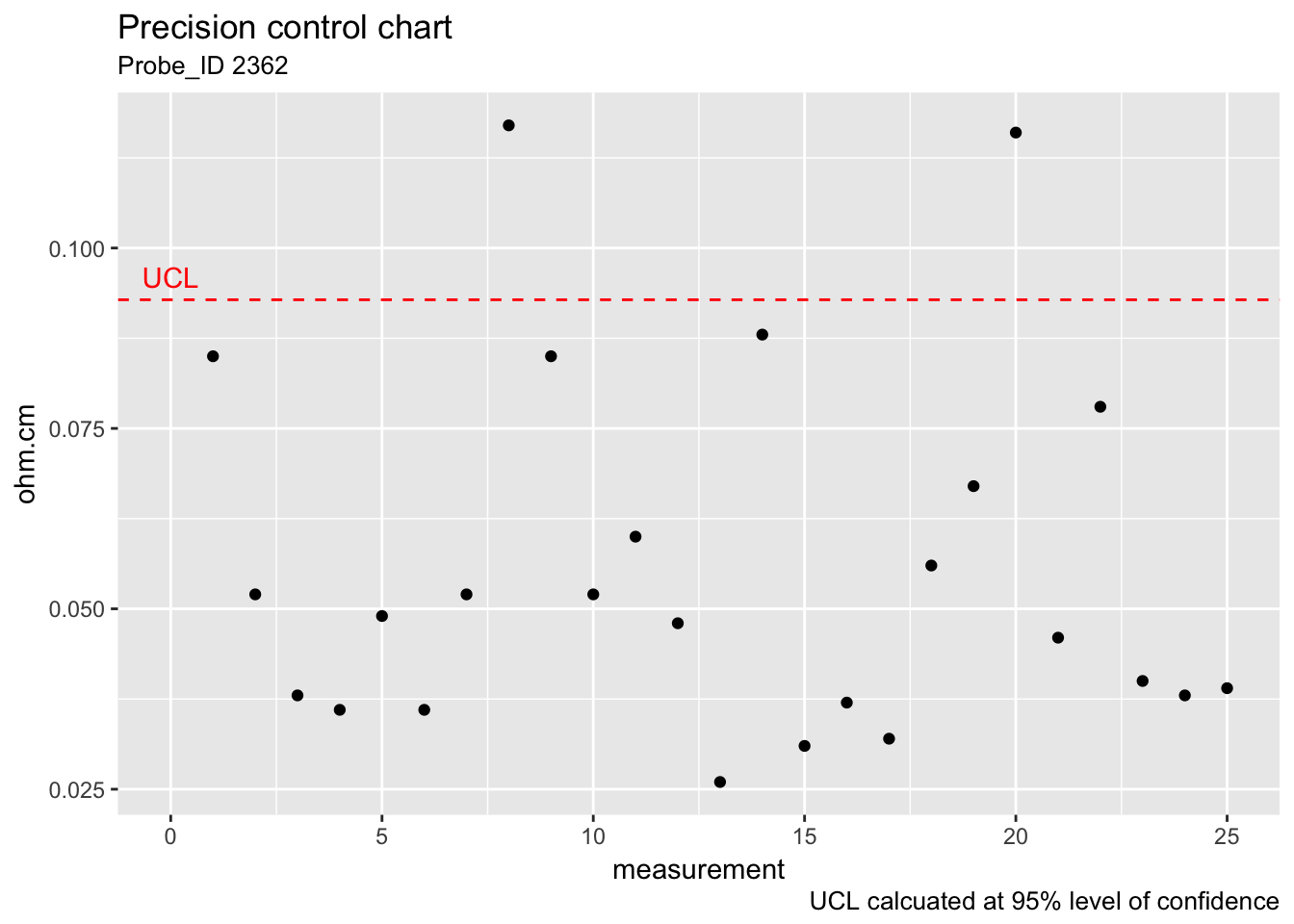#### 2.4.1.6 Control chart for measurement bias and variability The control limits for monitoring the bias and long-term variability of resistivity with a Shewhart control chart are given by $UCL=\text{Average} + 2 \cdot s_2 \\ Centerline=\text{Average} \\ LCL=\text{Average} − 2 \cdot s_2 \\$ ggplot(check_standard) + geom_point(aes(rowid, Resistance)) + geom_hline(aes(yintercept = (mean(Resistance) + 2*s2_chkstd)), colour = "red", linetype = "dashed") + geom_hline(aes(yintercept = (mean(Resistance) - 2*s2_chkstd)), colour = "red", linetype = "dashed") + labs(title = "Shewhart control chart", subtitle = "Probe_ID 2362", x = "measurement", y = "ohm.cm", caption = "Control limits calcuated with k = 2") + annotate("text", x = 0, y = 97.12, label = "UCL", colour = "red") + annotate("text", x = 0, y = 97.02, label = "LCL", colour = "red")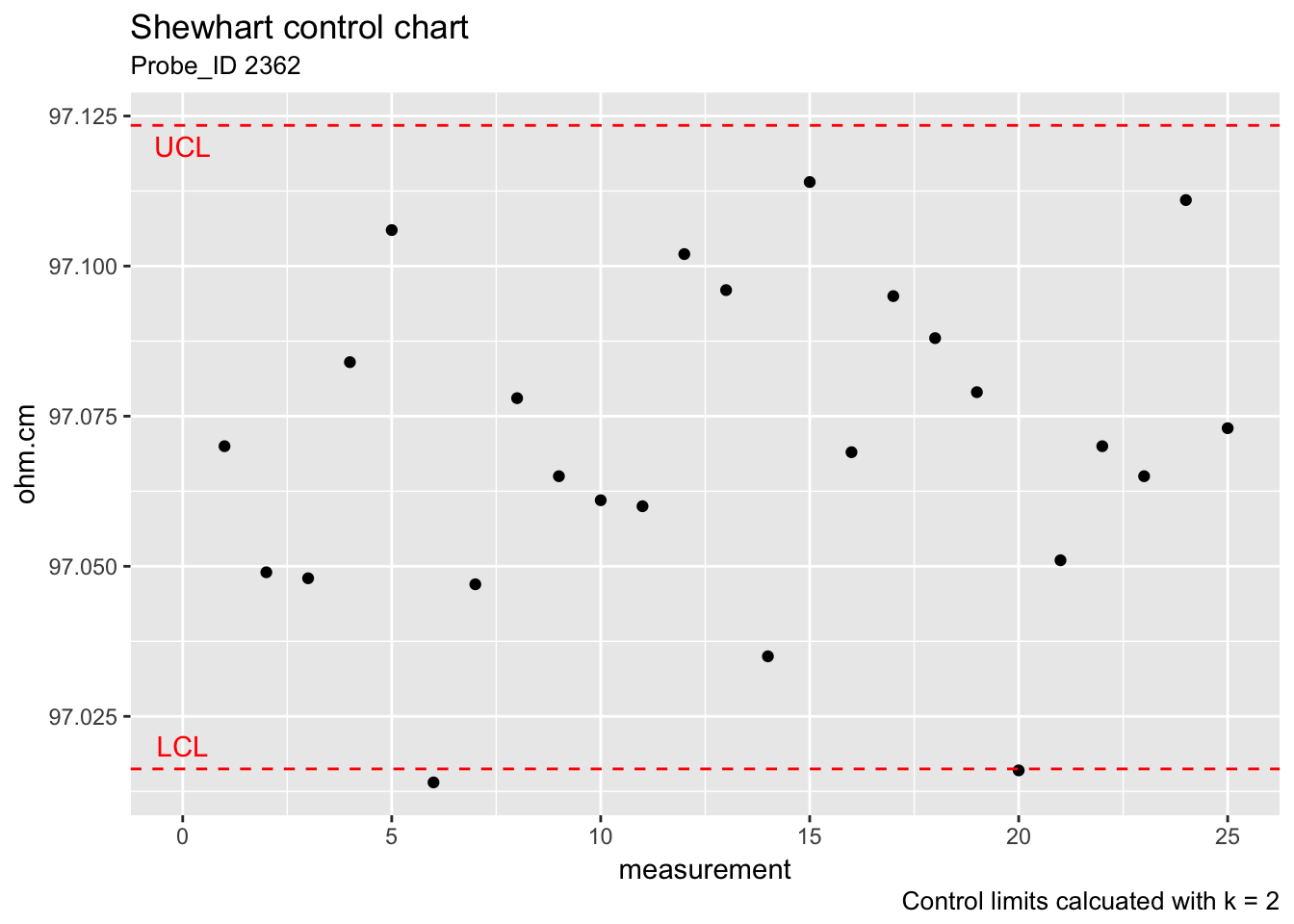### 2.4.2 Gauge study #### 2.4.2.1 Background and data Measurements on the check standards are used to estimate repeatability, day effect, and run effect The effect of operator was not considered to be significant for this study; therefore, ‘day’ replaces ‘operator’ as a factor in the nested design. Averages and standard deviations from J = 6 measurements at the center of each wafer are shown in the table. • J = 6 measurements at the center of the wafer per day • K = 6 days (one operator) per repetition • L = 2 runs (complete) • Q = 5 wafers (check standards 138, 139, 140, 141, 142) • R = 5 probes (1, 281, 283, 2062, 2362) Gauge study of resistivity probes gauge_study <- read_table2("NIST data/MPC61_clean.DAT", col_names = TRUE) %>% rowid_to_column() ## Parsed with column specification: ## cols( ## RUN = col_integer(), ## WAFER = col_double(), ## PROBE = col_double(), ## MONTH = col_double(), ## DAY = col_double(), ## OP = col_double(), ## TEMP = col_double(), ## AVERAGE = col_double(), ## STDDEV = col_double() ## ) gauge_study ## # A tibble: 300 x 10 ## rowid RUN WAFER PROBE MONTH DAY OP TEMP AVERAGE STDDEV ## <int> <int> <dbl> <dbl> <dbl> <dbl> <dbl> <dbl> <dbl> <dbl> ## 1 1 1 138. 1. 3. 15. 1. 23.0 95.2 0.119 ## 2 2 1 138. 1. 3. 17. 1. 23.0 95.2 0.0183 ## 3 3 1 138. 1. 3. 18. 1. 22.8 95.2 0.128 ## 4 4 1 138. 1. 3. 21. 1. 23.2 95.2 0.0398 ## 5 5 1 138. 1. 3. 23. 2. 23.2 95.1 0.0346 ## 6 6 1 138. 1. 3. 23. 1. 23.2 95.1 0.154 ## 7 7 1 138. 281. 3. 16. 1. 23.0 95.2 0.0963 ## 8 8 1 138. 281. 3. 17. 1. 23.0 95.1 0.0606 ## 9 9 1 138. 281. 3. 18. 1. 22.8 95.1 0.0842 ## 10 10 1 138. 281. 3. 21. 1. 23.3 95.1 0.0973 ## # ... with 290 more rows #### 2.4.2.2 Repeatability standard deviations ggplot(gauge_study) + geom_point(aes(as.factor(WAFER), STDDEV, colour = as.factor(DAY))) + facet_wrap(~ as.factor(PROBE), nrow = 1)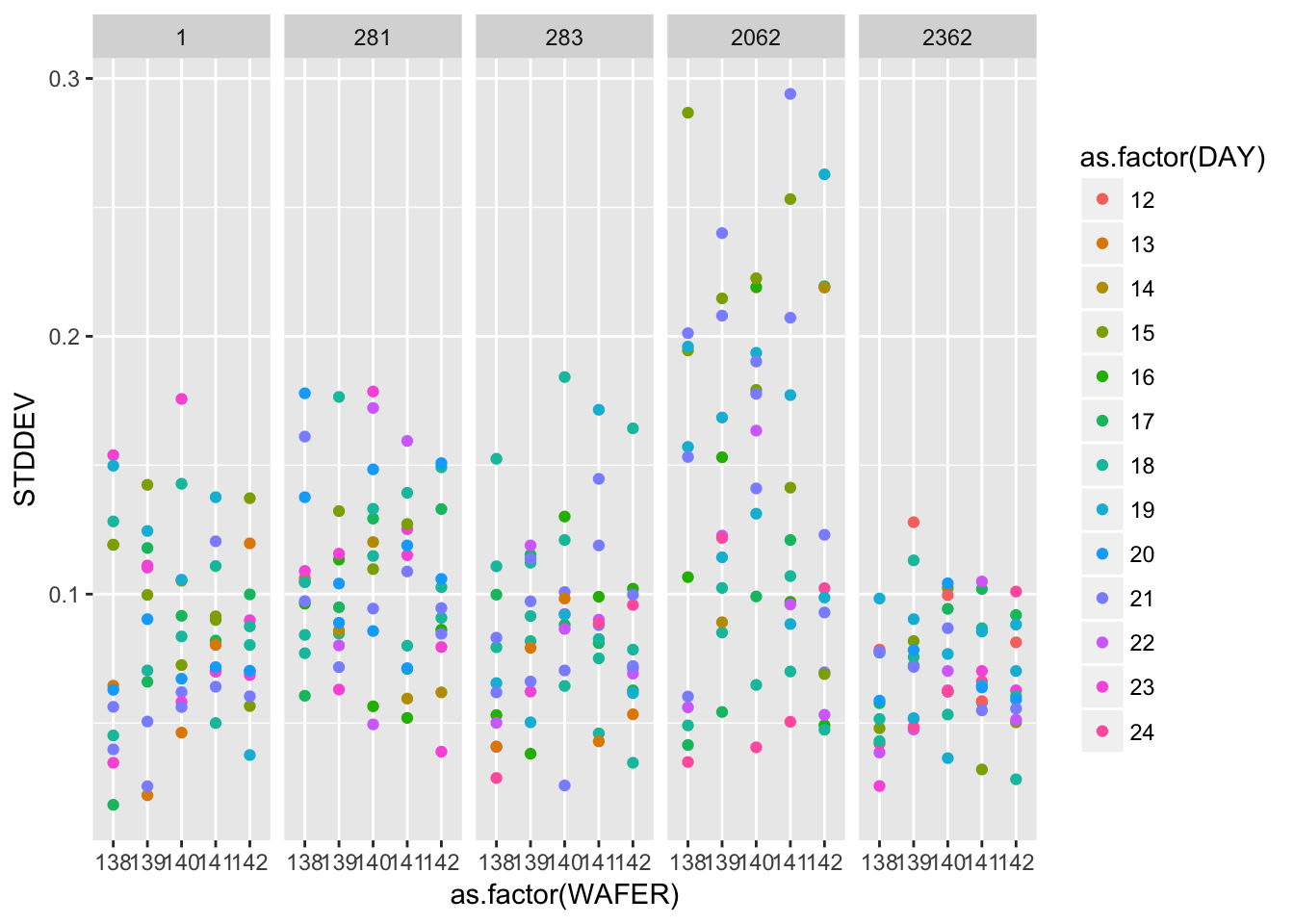ggplot(gauge_study) + geom_point(aes(as.factor(WAFER), STDDEV)) + facet_grid(as.factor(RUN) ~ as.factor(PROBE))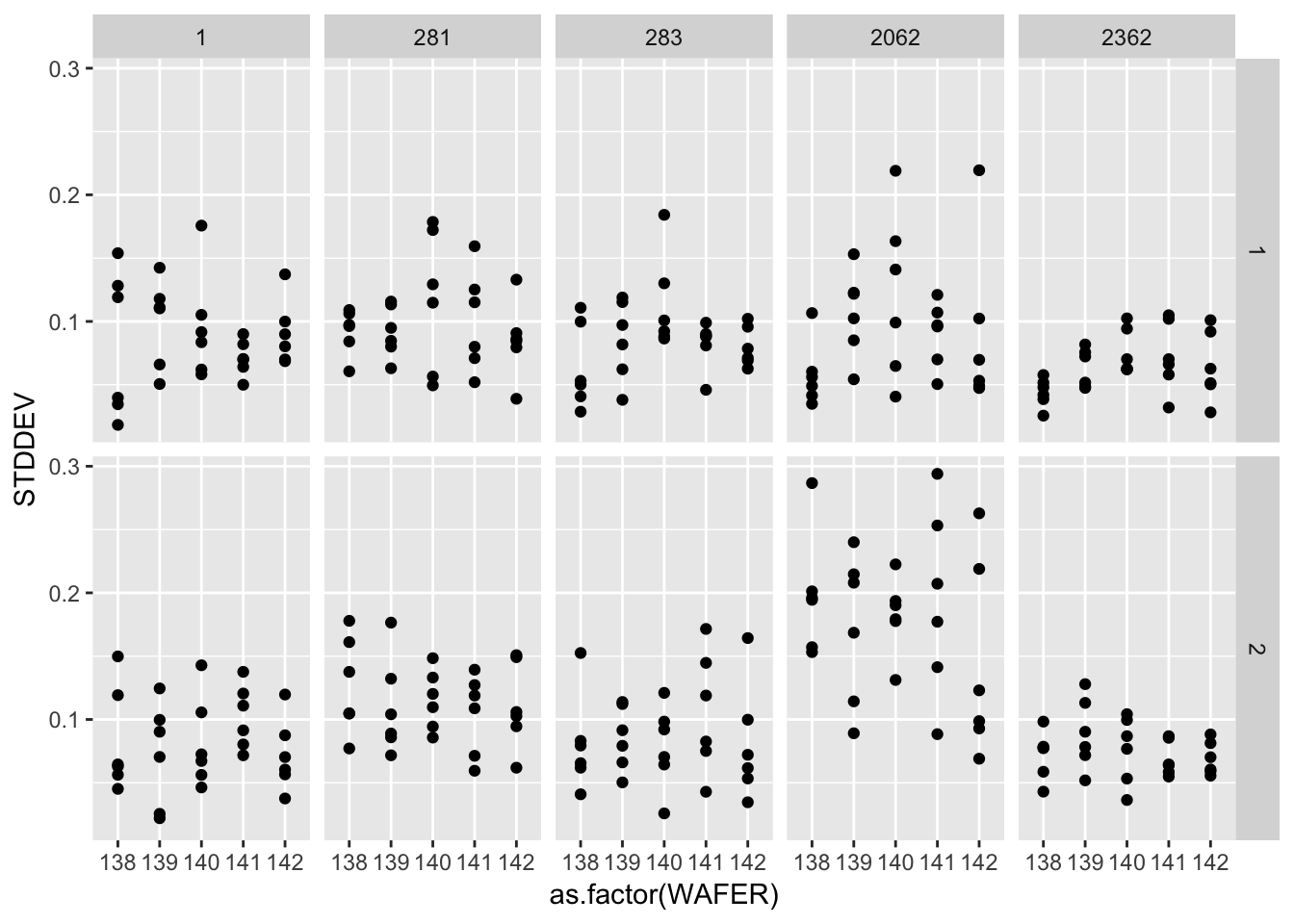#### 2.4.2.3 Effects of days and long-term stability ggplot(gauge_study) + geom_point(aes(rowid, AVERAGE, colour = as.factor(RUN))) + facet_grid(as.factor(WAFER) ~ as.factor(PROBE), scales = "free_y")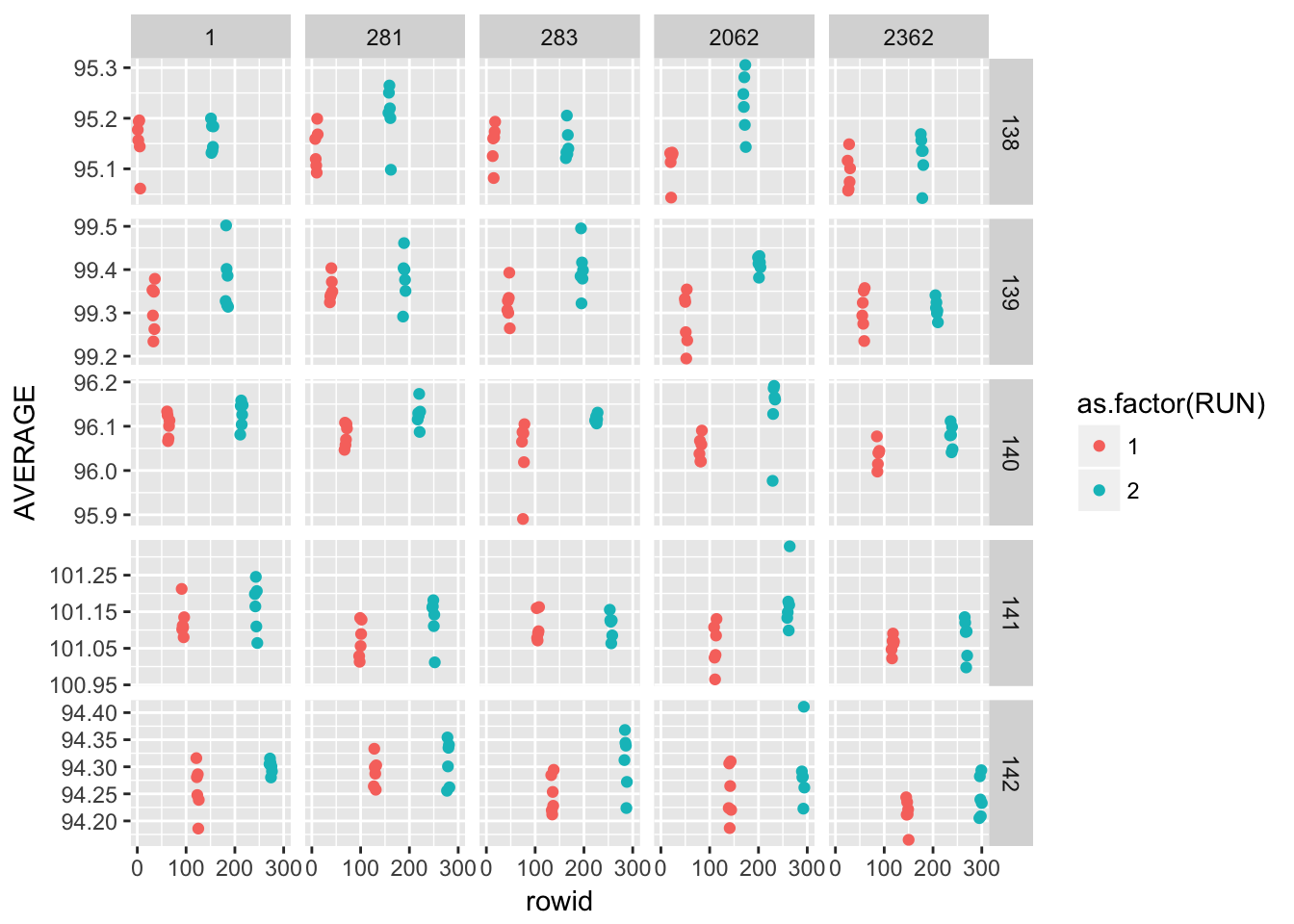#### 2.4.2.4 Differences among 5 probes probe_means_run <- gauge_study %>% group_by(PROBE, WAFER, RUN) %>% summarise(n = n(), probe_mean = mean(AVERAGE)) %>% unite(join_id, WAFER, RUN, sep = "_", remove = FALSE) %>% ungroup() probe_means_run ## # A tibble: 50 x 6 ## PROBE join_id WAFER RUN n probe_mean ## <dbl> <chr> <dbl> <int> <int> <dbl> ## 1 1. 138_1 138. 1 6 95.2 ## 2 1. 138_2 138. 2 6 95.2 ## 3 1. 139_1 139. 1 6 99.3 ## 4 1. 139_2 139. 2 6 99.4 ## 5 1. 140_1 140. 1 6 96.1 ## 6 1. 140_2 140. 2 6 96.1 ## 7 1. 141_1 141. 1 6 101. ## 8 1. 141_2 141. 2 6 101. ## 9 1. 142_1 142. 1 6 94.3 ## 10 1. 142_2 142. 2 6 94.3 ## # ... with 40 more rows wafer_means_run <- gauge_study %>% group_by(WAFER, RUN) %>% summarise(n = n(), wafer_means = mean(AVERAGE)) %>% unite(join_id, WAFER, RUN, sep = "_", remove = FALSE) %>% ungroup() wafer_means_run ## # A tibble: 10 x 5 ## join_id WAFER RUN n wafer_means ## <chr> <dbl> <int> <int> <dbl> ## 1 138_1 138. 1 30 95.1 ## 2 138_2 138. 2 30 95.2 ## 3 139_1 139. 1 30 99.3 ## 4 139_2 139. 2 30 99.4 ## 5 140_1 140. 1 30 96.1 ## 6 140_2 140. 2 30 96.1 ## 7 141_1 141. 1 30 101. ## 8 141_2 141. 2 30 101. ## 9 142_1 142. 1 30 94.3 ## 10 142_2 142. 2 30 94.3 delta_probes <- left_join(probe_means_run, wafer_means_run, by = "join_id") %>% mutate(delta_probes_wafer = probe_mean - wafer_means) delta_probes ## # A tibble: 50 x 11 ## PROBE join_id WAFER.x RUN.x n.x probe_mean WAFER.y RUN.y n.y ## <dbl> <chr> <dbl> <int> <int> <dbl> <dbl> <int> <int> ## 1 1. 138_1 138. 1 6 95.2 138. 1 30 ## 2 1. 138_2 138. 2 6 95.2 138. 2 30 ## 3 1. 139_1 139. 1 6 99.3 139. 1 30 ## 4 1. 139_2 139. 2 6 99.4 139. 2 30 ## 5 1. 140_1 140. 1 6 96.1 140. 1 30 ## 6 1. 140_2 140. 2 6 96.1 140. 2 30 ## 7 1. 141_1 141. 1 6 101. 141. 1 30 ## 8 1. 141_2 141. 2 6 101. 141. 2 30 ## 9 1. 142_1 142. 1 6 94.3 142. 1 30 ## 10 1. 142_2 142. 2 6 94.3 142. 2 30 ## # ... with 40 more rows, and 2 more variables: wafer_means <dbl>, ## # delta_probes_wafer <dbl> ggplot(delta_probes) + geom_line(aes(as.factor(WAFER.x), delta_probes_wafer, group = as.factor(PROBE), colour = as.factor(PROBE))) + geom_hline(aes(yintercept = 0), linetype = "dashed") + facet_wrap(~ as.factor(RUN.x), ncol = 1)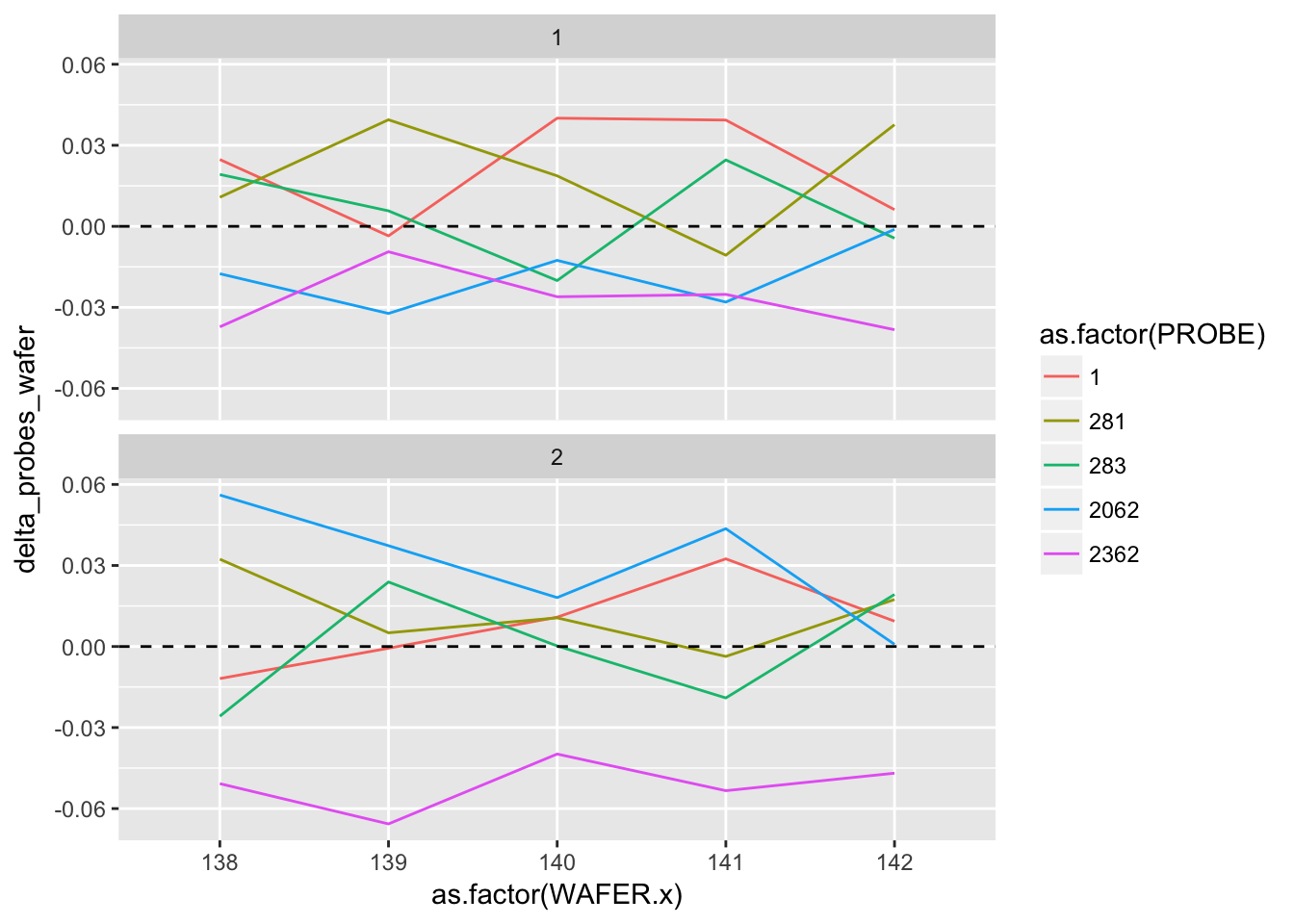#### 2.4.2.5 Analysis and interpretation Table of estimates for probe #2362 A graphical analysis shows repeatability standard deviations plotted by wafer and probe… The plots show that for both runs the precision of this probe is better than for the other probes. Probe #2362, because of its superior precision, was chosen as the tool for measuring all 100 ohm.cm resistivity wafers at NIST. Therefore, the remainder of the analysis focuses on this probe. #### 2.4.2.6 probe #2362 probe_2362 <- gauge_study %>% filter(PROBE == 2362) probe_2362 ## # A tibble: 60 x 10 ## rowid RUN WAFER PROBE MONTH DAY OP TEMP AVERAGE STDDEV ## <int> <int> <dbl> <dbl> <dbl> <dbl> <dbl> <dbl> <dbl> <dbl> ## 1 25 1 138. 2362. 3. 15. 1. 23.1 95.1 0.0480 ## 2 26 1 138. 2362. 3. 17. 1. 23.0 95.1 0.0577 ## 3 27 1 138. 2362. 3. 18. 1. 23.0 95.1 0.0516 ## 4 28 1 138. 2362. 3. 22. 1. 23.2 95.1 0.0386 ## 5 29 1 138. 2362. 3. 23. 2. 23.3 95.1 0.0256 ## 6 30 1 138. 2362. 3. 24. 2. 23.1 95.1 0.0420 ## 7 55 1 139. 2362. 3. 15. 1. 23.1 99.3 0.0818 ## 8 56 1 139. 2362. 3. 17. 1. 23.0 99.3 0.0723 ## 9 57 1 139. 2362. 3. 18. 1. 22.9 99.3 0.0756 ## 10 58 1 139. 2362. 3. 22. 1. 23.3 99.4 0.0475 ## # ... with 50 more rows Pooled level-1 standard deviations (ohm.cm) s1_2362_1 <- probe_2362 %>% filter(RUN == 1) %>% mutate(Stdev_sq = STDDEV^2) %$%
mean(Stdev_sq) %>%
sqrt()

s1_2362_1
##  0.06750898
s1_2362_2 <- probe_2362 %>%
filter(RUN == 2) %>%
mutate(Stdev_sq = STDDEV^2) %$% mean(Stdev_sq) %>% sqrt() s1_2362_2 ##  0.07785664 s1_2362 <- probe_2362 %>% mutate(Stdev_sq = STDDEV^2) %$%
mean(Stdev_sq) %>%
sqrt()

s1_2362
##  0.07286673

Level-2 standard deviations (ohm.cm) for 5 wafers

s2_2362 <- gauge_study %>%
group_by(PROBE, WAFER, RUN) %>%
filter(PROBE == 2362) %>%
summarise(df = n()-1, probe_mean = mean(AVERAGE), probe_stdev = sd(AVERAGE), probe_stdev_sq = probe_stdev^2) %>%
group_by(RUN) %>%
summarise(s2_run = sqrt(mean(probe_stdev_sq)))

s2_2362
## # A tibble: 2 x 2
##     RUN s2_run
##   <int>  <dbl>
## 1     1 0.0333
## 2     2 0.0388

Over both runs

s2_2352_all <- s2_2362 %>%
mutate(s2_run_sq = s2_run^2) %$% mean(s2_run_sq) %>% sqrt() s2_2352_all ##  0.03616824 sd_2362_wafer <- gauge_study %>% group_by(PROBE, WAFER, RUN) %>% filter(PROBE == 2362) %>% summarise(probe_mean = mean(AVERAGE)) %>% mutate( run_number = case_when( RUN == 1 ~ "Run1", RUN == 2 ~ "Run2" ) ) %>% dplyr::select(PROBE, WAFER, probe_mean, run_number) %>% group_by(WAFER) %>% summarise(sd_wafer = sd(probe_mean)) sd_2362_wafer ## # A tibble: 5 x 2 ## WAFER sd_wafer ## <dbl> <dbl> ## 1 138. 0.0222 ## 2 139. 0.00271 ## 3 140. 0.0288 ## 4 141. 0.0133 ## 5 142. 0.0205 s3_2362 <- sd_2362_wafer %>% mutate(sd_wafer_sq = sd_wafer^2) %$%
mean(sd_wafer_sq) %>%
sqrt()

s3_2362
##  0.01964524